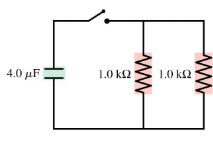# Problem: What is the time constant for the discharge of the capacitors in the figure (Figure 1)?

###### FREE Expert Solution

The time constant for the discharge of capacitor:

$\overline{){\mathbf{T}}{\mathbf{=}}{\mathbf{R}}{\mathbf{C}}}$

The resistors are in parallel.

$\overline{){{\mathbf{R}}}_{\mathbf{e}\mathbf{q}}{\mathbf{=}}\frac{{\mathbf{R}}_{\mathbf{1}}{\mathbf{R}}_{\mathbf{2}}}{{\mathbf{R}}_{\mathbf{1}}\mathbf{+}{\mathbf{R}}_{\mathbf{2}}}}$

95% (236 ratings)###### Problem Details
What is the time constant for the discharge of the capacitors in the figure (Figure 1)?